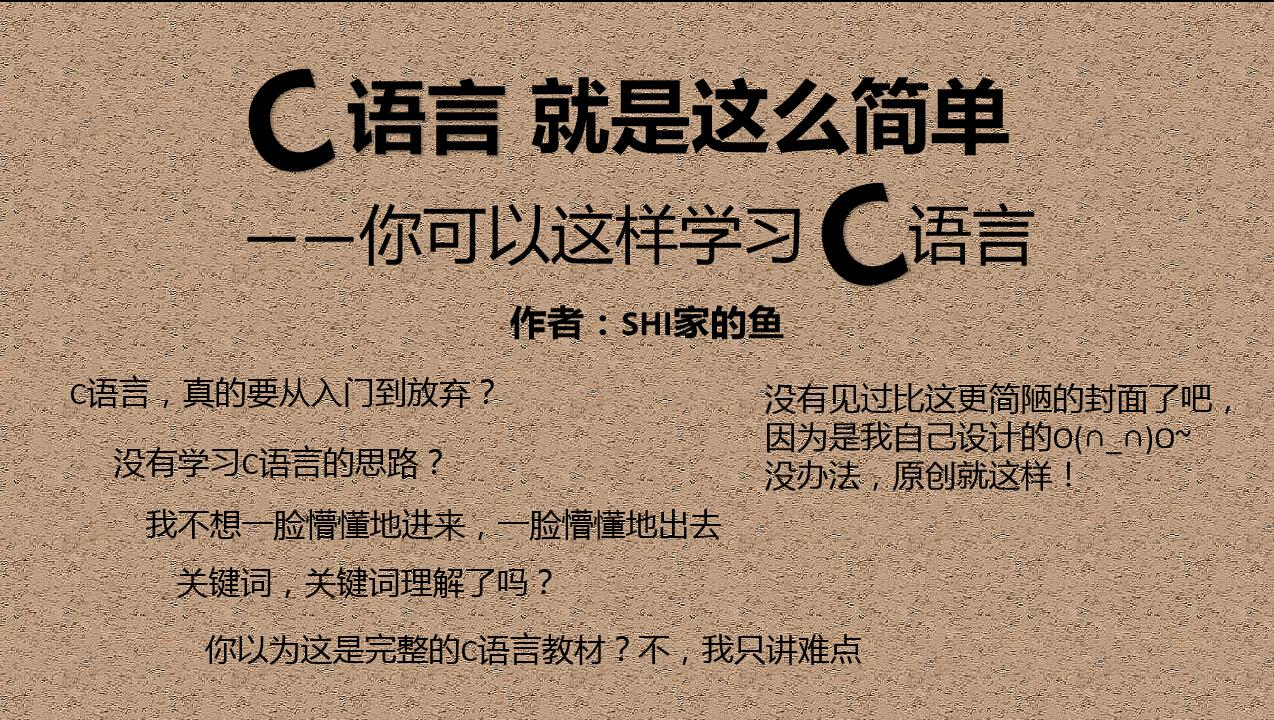1. 计算机基础知识的学习
2. C语言语法的学习
3. C程序编程练习
4. 计算机其他学科的学习
5. 工作业务的学习

## 三、学习C语言不妨试试这些技巧

### 3.1 发明自己的术语

``````#include <stdio.h>

int main(void)
{
int array = { {11, 12}, {21, 22}, {31, 32} };
int (*pArray) = NULL, index1 = 0, index2 = 0;

pArray = array;

for(index1 = 0; index1 < 3; index1++)
{
printf("The %dth line\n", index1 + 1);
for(index2 = 0; index2 < 2; index2++)
{
printf("%d ", *(*(pArray + index1) + index2) );
}
printf("\n");
}

return 0;
}``````

``*(*(pArray + index1) + index2)``

### 3.2 联想相关学科

C语言有函数概念，数学中也有函数概念，它们之间是有某种相似性的。如果你中学时数学函数学的好，那么你再学习C语言函数概念时你会发现好多东西都是已经学过的。这部分章节文章如下：